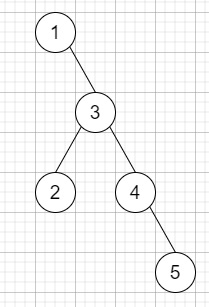# Binary Tree Longest Consecutive Sequence in C++

Suppose we have a binary tree; we have to check whether we can find the length of the longest consecutive sequence path. If the path refers to any sequence of nodes from some starting node to any node in the tree along the parent-child connections. The longest consecutive path need to follow parent to child but not reverse.

So, if the input is like,then the output will be 3, as the Longest consecutive sequence path is 3-4-5, so return 3.

To solve this, we will follow these steps −

• Define a function solveUtil(), this will take node, prev, len initialize it with 1,

• if node is null, then −

• return

• if prev + 1 is same as val of node, then −

• (increase len by 1)

• ans := maximum of ans and len

• solveUtil(left of node, val of node, len)

• solveUtil(right of node, val of node, len)

• Otherwise

• solveUtil(left of node, val of node, 1)

• solveUtil(right of node, val of node, 1)

• Define a function solve(), this will take A,

• ans := 1

• solveUtil(A, -infinity)

• return ans

• From the main method do the following −

• if root is null, then −

• return 0

• return solve(root)

## Example

Let us see the following implementation to get a better understanding −

Live Demo

#include <bits/stdc++.h>
using namespace std;
class TreeNode{
public:
int val;
TreeNode *left, *right;
TreeNode(int data){
val = data;
left = NULL;
right = NULL;
}
};
void insert(TreeNode **root, int val){
queue<TreeNode*> q;
q.push(*root);
while(q.size()){
TreeNode *temp = q.front();
q.pop();
if(!temp->left){
if(val != NULL)
temp->left = new TreeNode(val);
else
temp->left = new TreeNode(0);
return;
}else{
q.push(temp->left);
}
if(!temp->right){
if(val != NULL)
temp->right = new TreeNode(val);
else
temp->right = new TreeNode(0);
return;
}else{
q.push(temp->right);
}
}
}
TreeNode *make_tree(vector<int< v){
TreeNode *root = new TreeNode(v);
for(int i = 1; i<v.size(); i++){
insert(&root, v[i]);
}
return root;
}
class Solution {
public:
int ans;
void solveUtil(TreeNode* node, int prev, int len = 1){
if (!node)
return;
if (prev + 1 == node->val) {
len++;
ans = max(ans, len);
solveUtil(node->left, node->val, len);
solveUtil(node->right, node->val, len);
}
else {
solveUtil(node->left, node->val, 1);
solveUtil(node->right, node->val, 1);
}
}
int solve(TreeNode* A){
ans = 1;
solveUtil(A, INT_MIN);
return ans;
}
int longestConsecutive(TreeNode* root){
if (!root)
return 0;
return solve(root);
}
};
main(){
Solution ob;
TreeNode *root = new TreeNode(1);
root->right = new TreeNode(3);
root->right->left = new TreeNode(2);
root->right->right = new TreeNode(4);
root->right->right->right = new TreeNode(5);
cout << (ob.longestConsecutive(root));
}

## Input

TreeNode *root = new TreeNode(1);
root->right = new TreeNode(3);
root->right->left = new TreeNode(2);
root->right->right = new TreeNode(4);
root->right->right->right = new TreeNode(5);

## Output

3